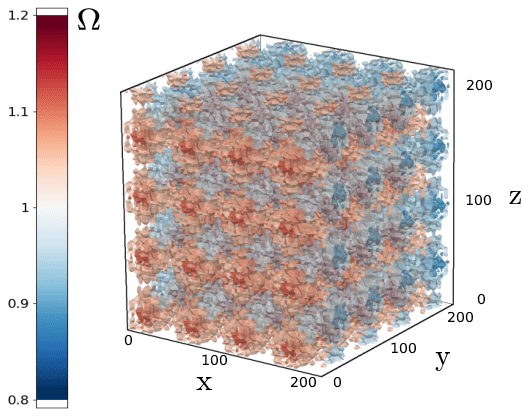Research topics

• Mathematical formulations of gravitational physics
• Lie symmetry analysis and its application to astrophysics
• The theory of structure formation in the early Universe
• Gravitational lensing in cosmology
• Chaos and critical phenomena in General Relativity
• Computer algebra systems with application to general relativity
• Gravitational waves in General Relativity
•  The first detection of gravitational waves in 2015 opened a new window on the Universe. Gravitational waves which approach Earth-based detectors are extremely weak and, as such, very well described by the linearized Einstein's gravity theory. If full Einstein's equations are taken into account new phenomena come into play. This 'territory' is mainly unexplored and is a subject of mathematical studies conducted in our department: Sebastian J. Szybka, Syed U. NaqviFreely falling bodies in a standing wave space-time Sebastian J. Szybka, Adam CieślikStanding waves in general relativityOne of the metric functions of the Einstein-Rosen gravitational waves.
• Inhomogeneous cosmology
•  In the standard cosmological model it is assumed that the matter is distributed homogeneously in space. Although this model is consistent with a variety of astronomical observations, it brings us to some mysteries. To achieve the agreement between the model predictions and the observations one should introduce the cosmological constant - the term in the Einstein equations connected with the hypothetical form of matter called dark energy, which physical nature is unknown. In our department we study the more general class of cosmological models, which admit the existence of matter inhomogeneities representing the large-scale structures in the Universe. We study how these inhomogeneities could influence the cosmological observations. Some of our recent results on this topic are: Krzysztof Głód1+1+2 covariant formulation of light propagation in spacetime Szymon Sikora, Krzysztof GłódPerturbatively constructed cosmological model with periodically distributed dust inhomogeneitiesThe matter distribution in space of the one of the considered cosmological models.